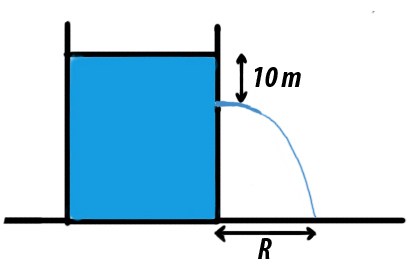# Hole in the Wall!A large tank is filled with water (density $\rho$). A small hole is made at a depth of $h = 10m$ below the water surface. The range of water issuing out of the hole is $R$ on ground. What Extra Pressure (in atm) must be applied on the water surface so that the range becomes $2R$?

Details and Assumptions:

$\bullet$ The answer to this problem is an Integer
$\bullet \rho = 10^3 kg/m^3$
$\bullet g = 10 m/s^2$
$\bullet$ Water is assumed to be ideal liquid

×

Problem Loading...

Note Loading...

Set Loading...